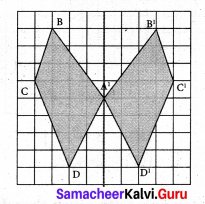# Samacheer Kalvi 7th Maths Solutions Term 3 Chapter 4 Geometry Intext Questions

Students can Download Maths Chapter 4 Geometry Intext Questions and Answers, Notes Pdf, Samacheer Kalvi 7th Maths Book Solutions Guide Pdf helps you to revise the complete Tamilnadu State Board New Syllabus and score more marks in your examinations.

## Tamilnadu Samacheer Kalvi 7th Maths Solutions Term 3 Chapter 4 Geometry Intext Questions

Exercise 4.1
Try These (Text book Page No. 72)

Question 1.
Can you draw a shape which has no line of symmetry?
Solution:
Yes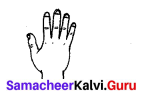Question 2.
Draw all possible line of symmetry for the following shapes.
Solution: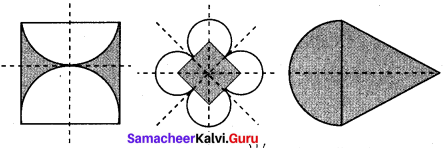Think (Text book Page No. 73)

Question 1.
What can you say about the number of lines of symmetry of a circle?
Solution:
A circle has infinite number of lines of symmetry.

Try These (Text book Page No. 73)

Question 1.
Reflect the words CHEEK, BIKE, BOX with horizontal line.
Solution: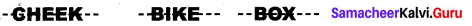Question 2.
Reflect the following words with vertical line.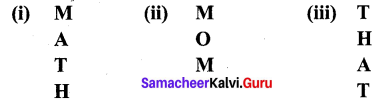Solution: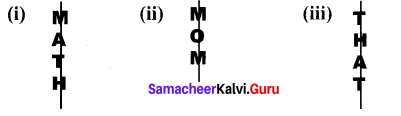Think (Textbook Page no.73)

Question 1.
Will the figure be symmetric about both the diagonals?
Solution:
Yes, it is symmetric about both the diagonals.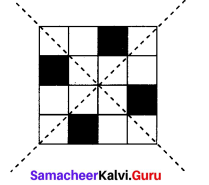Try These (Text book Page No. 74)

Question 1.
Find the order of rotational symmetry of the following figures.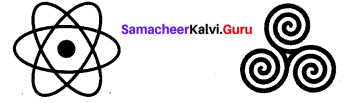Solution:
Order of symmetry : 6
Order of symmetry : 3

Question 2.
Find the order of rotational symmetry for an equilateral triangle.
Solution: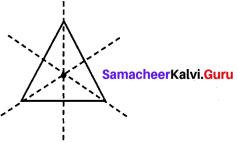For an equilateral triangle order of rotational symmetry is 3.Think (Text book Page No. 74)

Question 1.
Can a parallelogram have a rotational symmetry?
Solution: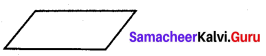Yes, order of rotational symmetry is 2.

Try These (Text book Page No. 75)

Question 1.
Using translational symmetry make new pattern with the given figure.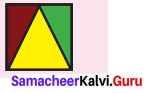Solution: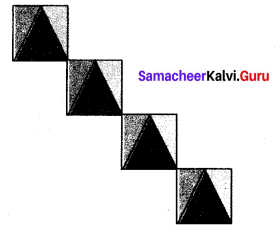Try These (Text book Page No. 77)

Question 1.
Translate this figure to 4 → 3↑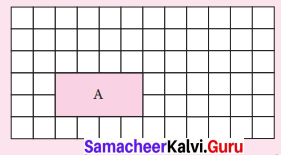Solution: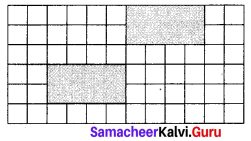Question 2.
Translate this figure to 2 ↓ 1 ←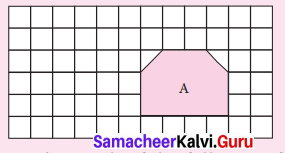Solution: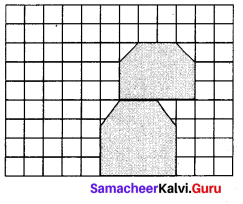Question 3.
How is the pre-image A translated to image A’ in each of the following figures?
(i)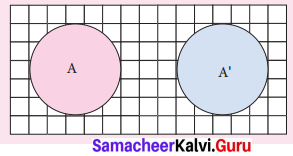(ii)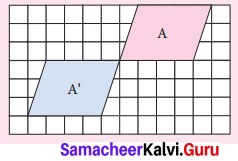Solution:
(i) 8 →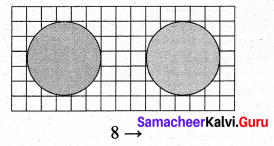(ii) 5 → 3 ↑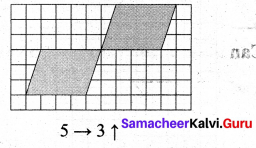Think (Text book Page No. 78)

Question 1.
The pre-image and the image after a translation coincide. What can you say about the translation
Solution:
There is no right, left, up or down movement took place.

Try These (Text book Page No. 80)

Question 1.
Draw the line reflection in the following pictures.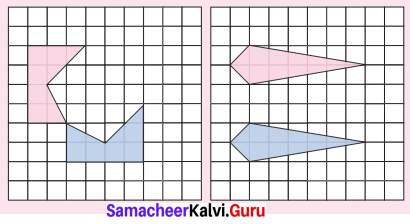Solution: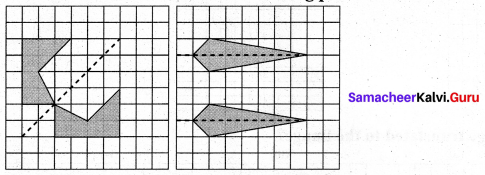Question 2.
Reflect the shape with given line of reflection.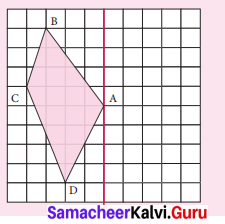Solution: